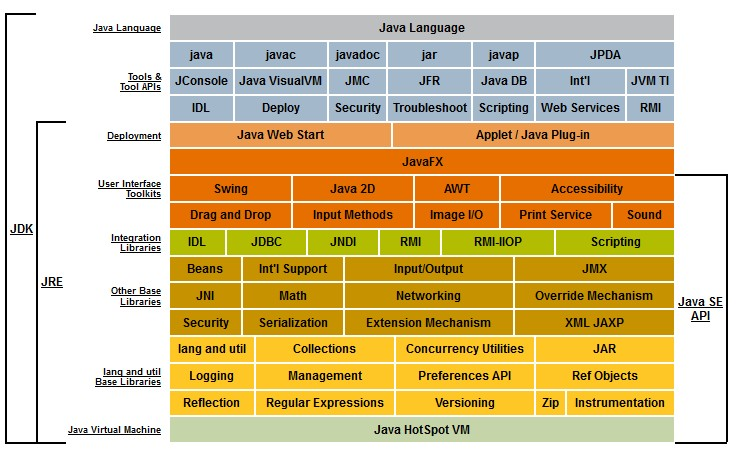# Java - Generic Class

generic class

All instances of a generic class have the same run-time class, regardless of their actual type parameters.

``````List<String> l1 = new ArrayList<String>();
List<Integer> l2 = new ArrayList<Integer>();
System.out.println(l1.getClass() == l2.getClass());```
```
``````true
```
```

A class generic has the same behavior for all of its possible type parameters; the same class can be viewed as having many different types.

The class java.lang.Class is generic. It has a type parameter T. Class

'' - The type of String.class is Class
Articles Related
Syntax

A generic class is defined with the following format:

````class name<T1, T2, ..., Tn> { /* ... */ }`
```

where:

• the angle brackets (Diamond) (<>) is the type parameter section
• T1, T2, …, and Tn are the type parameters (also called type variables). T stands for “Type”. The type variable, T, that can then be used anywhere inside the class.
Initialization
``````Box<Integer> integerBox = new Box<Integer>();
Box<Integer> integerBox = new Box<>(); // The parameters are optional after the new keyword because it can be inferred.
// Use Class<?> if the class being modeled is unknown.```
```
Raw Type

If the actual type argument is omitted, you create a raw type of Box

:
``````Box rawBox = new Box();
// Same as
Box<Object> rawBox = new Box<>()```
```

If you assign a raw type to a parameterized type, you get a warning:

``````Box rawBox = new Box();           // rawBox is a raw type of Box<T>
Box<Integer> intBox = rawBox;     // warning: unchecked conversion```
```
Example
Class

A Generic version of a class ClassName. The @param

is the type of the value being boxed
``````public class ClassName<T> {

// T stands for "Type"
private T t;
public void set(T t) { this.t = t; }
public T get() { return t; }

}```
```

Discover MoreDesign Pattern - Typesafe heterogeneous container (Java)

Typesafe heterogeneous container pattern. Container meaning a list of objects. A container instance is: typesafe: when it will never return an Integer when you ask it for a String. heterogenous:...Java - (Generic|Parameterized) type - (Class|Interface|Method) Parametrization

and Integer.class is of type Class Stronger type checks at compile time. Fixing compile-time errors is easier than fixing runtime errors, which can be difficult to find. The compiler can check the type...